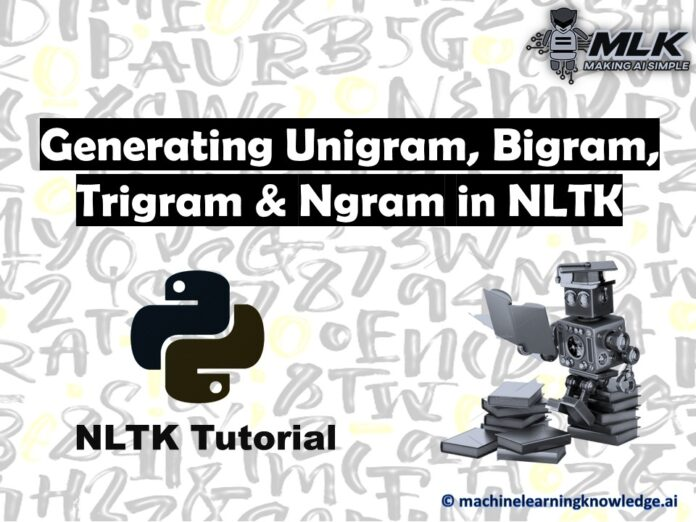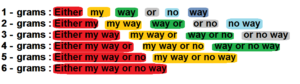# Generating Unigram, Bigram, Trigram and Ngrams in NLTKIn this tutorial, we will understand the concept of ngrams in NLP and why it is used along with its variations like Unigram, Bigram, Trigram. Then we will see examples of ngrams in NLTK library of Python and also touch upon another useful function everygram. So let us begin.

## What is n-gram Model

In natural language processing n-gram is a contiguous sequence of n items generated from a given sample of text where the items can be characters or words and n can be any numbers like 1,2,3, etc.

For example, let us consider a line – “Either my way or no way”, so below is the possible n-gram models that we can generate –As we can see using the n-gram model we can generate all possible contiguous combinations of length n for the words in the sentence. When n=1, the n-gram model resulted in one word in each tuple. When n=2, it generated 5 combinations of sequences of length 2, and so on.

Similarly for a given word we can generate n-gram model to create sequential combinations of length n for characters in the word. For example from the sequence of characters “Afham”, a 3-gram model will be generated as “Afh”, “fha”, “ham”, and so on.

Due to their frequent uses, n-gram models for n=1,2,3 have specific names as Unigram, Bigram, and Trigram models respectively.

## Use of n-grams in NLP

• N-Grams are useful to create features from text corpus for machine learning algorithms like SVM, Naive Bayes, etc.
• N-Grams are useful for creating capabilities like autocorrect, autocompletion of sentences, text summarization, speech recognition, etc.

## Generating ngrams in NLTK

We can generate ngrams in NLTK quite easily with the help of ngrams function present in nltk.util module. Let us see different examples of this NLTK ngrams function below.

### Unigrams or 1-grams

To generate 1-grams we pass the value of n=1 in ngrams function of NLTK. But first, we split the sentence into tokens and then pass these tokens to ngrams function.

As we can see we have got one word in each tuple for the Unigram model.

In :
```from nltk.util import ngrams

n = 1
sentence = 'You will face many defeats in life, but never let yourself be defeated.'
unigrams = ngrams(sentence.split(), n)

for item in unigrams:
print(item)
```
[Out] :
```('You',)
('will',)
('face',)
('many',)
('defeats',)
('in',)
('life,',)
('but',)
('never',)
('let',)
('yourself',)
('be',)
('defeated.',)```

### Bigrams or 2-grams

For generating 2-grams we pass the value of n=2 in ngrams function of NLTK. But first, we split the sentence into tokens and then pass these tokens to ngrams function.

As we can see we have got two adjacent words in each tuple in our Bigrams model.

In :
```from nltk.util import ngrams

n = 2
sentence = 'The purpose of our life is to happy'
unigrams = ngrams(sentence.split(), n)

for item in unigrams:
print(item)
```
[Out] :
```('The', 'purpose')
('purpose', 'of')
('of', 'our')
('our', 'life')
('life', 'is')
('is', 'to')
('to', 'happy')```

### Trigrams or 3-grams

In case of 3-grams, we pass the value of n=3 in ngrams function of NLTK. But first, we split the sentence into tokens and then pass these tokens to ngrams function.

As we can see we have got three words in each tuple for the Trigram model.

In :
```from nltk.util import ngrams

n = 3
sentence = 'Whoever is happy will make others happy too'
unigrams = ngrams(sentence.split(), n)

for item in unigrams:
print(item)
```
[Out] :
```('Whoever', 'is', 'happy')
('is', 'happy', 'will')
('happy', 'will', 'make')
('will', 'make', 'others')
('make', 'others', 'happy')
('others', 'happy', 'too')```

## Generic Example of ngram in NLTK

In the example below, we have defined a generic function ngram_convertor that takes in a sentence and n as an argument and converts it into ngrams.

In :
```from nltk.util import ngrams
def ngram_convertor(sentence,n=3):

ngram_sentence = ngrams(sentence.split(), n)
for item in ngram_sentence:
print(item)
```
In :
```sentence = "Life is either a daring adventure or nothing at all"
ngram_convertor(sentence,3)
```
[Out] :
```('Life', 'is', 'either')
('is', 'either', 'a')
('either', 'a', 'daring')
('or', 'nothing', 'at')
('nothing', 'at', 'all')```

## NLTK Everygrams

NTK provides another function everygrams that converts a sentence into unigram, bigram, trigram, and so on till the ngrams, where n is the length of the sentence. In short, this function generates ngrams for all possible values of n.

Let us understand everygrams with a simple example below. We have not provided the value of n, but it has generated every ngram from 1-grams to 5-grams where 5 is the length of the sentence, hence the name everygram.

In :
```from nltk.util import everygrams

message = "who let the dogs out"
msg_split = message.split()

list(everygrams(msg_split))
```
[Out] :
```[('who',),
('let',),
('the',),
('dogs',),
('out',),
('who', 'let'),
('let', 'the'),
('the', 'dogs'),
('dogs', 'out'),
('who', 'let', 'the'),
('let', 'the', 'dogs'),
('the', 'dogs', 'out'),
('who', 'let', 'the', 'dogs'),
('let', 'the', 'dogs', 'out'),
('who', 'let', 'the', 'dogs', 'out')]```

## Converting data frames into Trigrams

In this example, we will show you how you can convert a dataframes of text into Trigrams using the NLTK ngrams function.

In :
```import pandas as pd
[Out] :
0 Southern European bond yields hit multi-week lows
1 BRIEF-LG sells its entire stake in unit LG Lif…
2 BRIEF-Golden Wheel Tiandi says unit confirms s…
3 BRIEF-Sunshine 100 China Holdings Dec contract…
4 Euro zone stocks start 2017 with new one-year …
In :
```from nltk.util import ngrams
def ngramconvert(df,n=3):
for item in df.columns:
df['new'+item]=df[item].apply(lambda sentence: list(ngrams(sentence.split(), n)))
return df
```
In :
```new_df = ngramconvert(df,3)
```
Out:
0 Southern European bond yields hit multi-week lows [(Southern, European, bond), (European, bond, …
1 BRIEF-LG sells its entire stake in unit LG Lif… [(BRIEF-LG, sells, its), (sells, its, entire),…
2 BRIEF-Golden Wheel Tiandi says unit confirms s… [(BRIEF-Golden, Wheel, Tiandi), (Wheel, Tiandi…
3 BRIEF-Sunshine 100 China Holdings Dec contract… [(BRIEF-Sunshine, 100, China), (100, China, Ho…
4 Euro zone stocks start 2017 with new one-year … [(Euro, zone, stocks), (zone, stocks, start), …

## (Bonus) Ngrams in Textblob

Textblob is another NLP library in Python which is quite user-friendly for beginners. Below is an example of how to generate ngrams in Textblob

In :
```from textblob import TextBlob

data = 'Who let the dog out'
num = 3

n_grams = TextBlob(data).ngrams(num)
for grams in n_grams:
print(grams)
```
[Out] :
```['Who', 'let', 'the']
['let', 'the', 'dog']
['the', 'dog', 'out']```